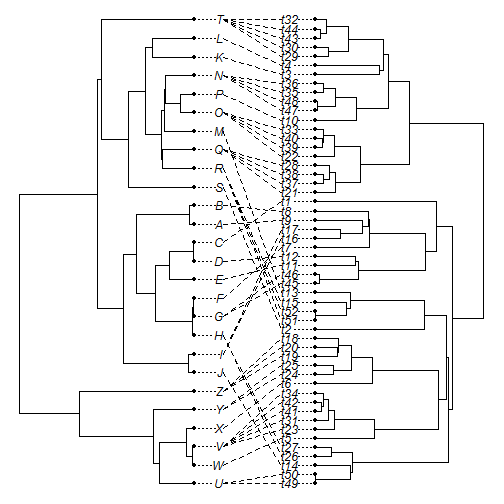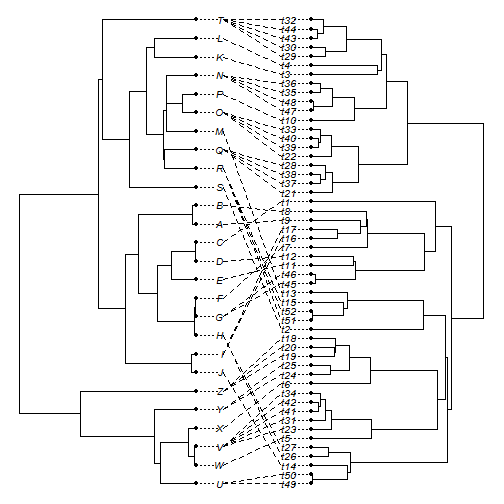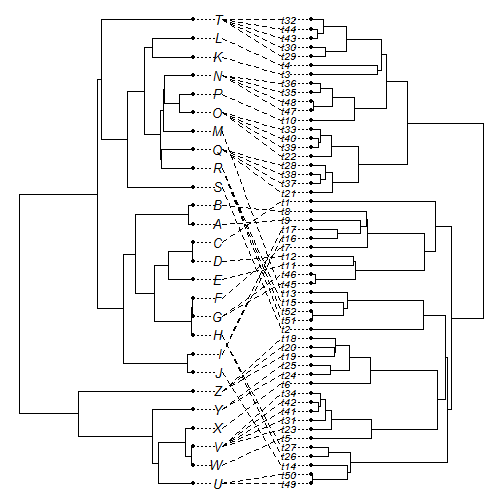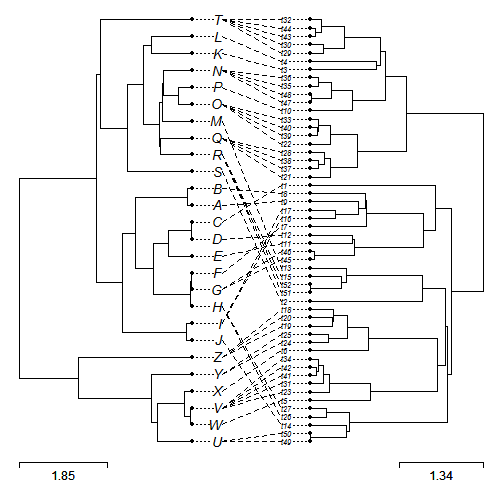## Saturday, April 23, 2016

### Control of font sizes in plotting an object of class `"cophylo"` with phytools

I received a user request recently about changing the font size in a plotted object of class `"cophylo"`. This is straightforward using the optional argument `fsize`, which is passed to the phytools to internal function `phylogram`. This is different from the more standard `cex` (character expansion factor); however it is kind of a legacy attribute of phytools' plotting functions, which are many.

When I saw his plotted `"cophylo"` object, however, it was apparent to me that the problem was not merely that he didn't know how to control font size, but that the font sizes of the two plotted phylogenies needed to be able to be controlled independently. This is because it is allowed to plot trees in which the right tree & the left tree have different numbers of tips.

The solution to this is a little bit more complicated than it might seem because the optional arguments are passed internally in `plot.cophylo` using the three-dot `...` argument. This makes it a little bit harder than it otherwise might be because we first have to put the `...` arguments in a list, then we can check the length of the list vector `fsize` (if it exists), then we can supply the first or second of these numeric values to the first or second call of `phylogram`, respectively, by modifying our argument list values & then calling the function with a list of arguments using the R base function `do.call`.

Here is a code-chunk to sort of illustrate what that looks like:

``````obj<-list(...)
## ...
leftArgs<-rightArgs<-obj
if(!is.null(obj\$fsize)){
if(length(obj\$fsize)>1){
leftArgs\$fsize<-obj\$fsize
rightArgs\$fsize<-obj\$fsize
## ...
}
}
x1<-do.call("phylogram",c(list(tree=x\$trees[],part=0.4),leftArgs))
## ...
x2<-do.call("phylogram",c(list(tree=x\$trees[],part=0.4,
direction="leftwards"),rightArgs))
``````

More details of the updates can be seen here and here.

The following is a quick demo to illustrate its application using simulated data & trees:

``````library(phytools)
t1
``````
``````##
## Phylogenetic tree with 26 tips and 25 internal nodes.
##
## Tip labels:
##  A, B, C, D, E, F, ...
##
## Rooted; includes branch lengths.
``````
``````t2
``````
``````##
## Phylogenetic tree with 52 tips and 51 internal nodes.
##
## Tip labels:
##  t16, t17, t9, t8, t7, t45, ...
##
## Rooted; includes branch lengths.
``````
``````head(assoc) ## top of association matrix
``````
``````##    [,1] [,2]
## I1 "I"  "t16"
## I2 "I"  "t17"
## A  "A"  "t9"
## B  "B"  "t8"
## F  "F"  "t7"
## G1 "G"  "t45"
``````
``````obj<-cophylo(t1,t2,assoc)
``````
``````## Rotating nodes to optimize matching...
## Done.
``````
``````obj
``````
``````## Object of class "cophylo" containing:
##
## (1) 2 (possibly rotated) phylogenetic trees in an object of class "multiPhylo".
##
## (2) A table of associations between the tips of both trees.
``````

Now if we plot using a single font size, then we either have text that is too small in one tree or too big in the other, for instance:

``````plot(obj) ## fsize=1
````````````plot(obj,fsize=0.8)
``````Let's try with two different font sizes:

``````plot(obj,fsize=c(1.1,0.8))
``````We can even add a scale bar with the same or different font size:

``````h1<-max(nodeHeights(t1))
h2<-max(nodeHeights(t2))
plot(obj,fsize=c(1.2,0.7,1),scale.bar=round(0.5*c(h1,h2),2))
``````Neat.

This version of phytools can be installed from GitHub as follows:

``````library(devtools)
install_github("liamrevell/phytools")
``````

The trees & associations were simulated as follows:

``````library(phangorn)
t1<-pbtree(n=26,tip.label=LETTERS)
t2<-pbtree(n=52)
tmp<-ltt(t2,plot=FALSE)
h<-tmp\$times[tmp\$ltt==26]
nn<-sapply(treeSlice(t2,h,trivial=TRUE),function(x) x\$tip.label)
N<-sapply(nn,length)
mm<-mapply(function(x,n) rep(x,n),
1.